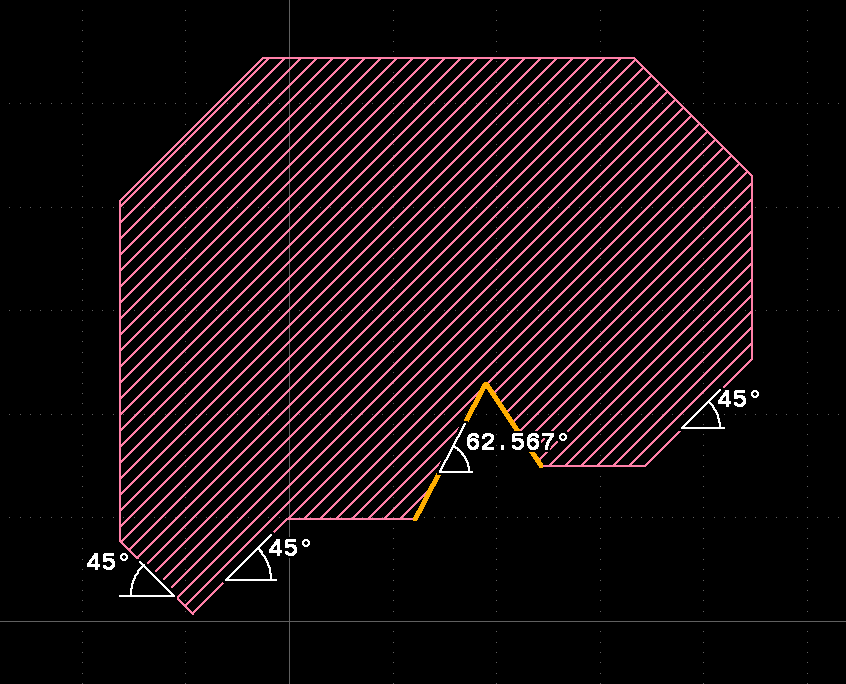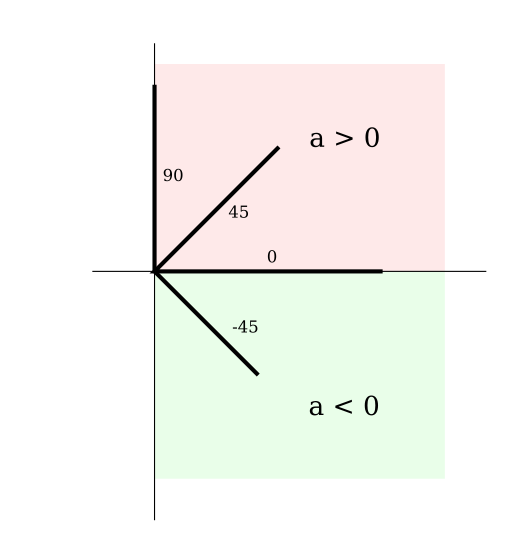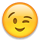# DRC to check 45degree angle shapes

The DRC needs to find non-45 degree shapes for Metal, so I coded this way :

``````(M1.edges - M1.edges.with_angle(0) - M1.edges.with_angle(45) - M1.edges.with_angle(90) - M1.edges.with_angle(135) - M1.edges.with_angle(180) - M1.edges.with_angle(225) - M1.edges.with_angle(270) - M1.edges.with_angle(315)).output("M1_angle", "Non 45 degree angle ME1")
``````

If I missed with_angles(225), I missed some errors. But is there a better and simpler way to code it ?

Laurent

• edited November 2022

Hi Laurent,

I'd suggest this solution:

``````e = M1.edges
[0, 90, -45, 45].each do |a|
e = e.without_angle(a)
end
e.output("M1_angle", "Non 45 degree angle ME1")
``````You don't need 135, 180 etc. angle checks. Angles are measured in a `]-90..90]` degree range, so -45 and 45 are enough to check for 45 edges with positive and negative slope:The solution with "without_angle" is somewhat more efficient as it avoids boolean operations. I also assume that the first two steps quickly eliminate most of the edges leaving only few for the 45 and -45 degree analysis.

One more remark from my side: when your code is like

``````M1.edges - M1.edges.with_angle(0) - M1.edges.with_angle(45) - ...
``````

"M1.edges" will be executed multiple times. It is not a very expensive operation, yet it can be avoided by computing the edges first:

``````M1_edges = M1.edges
M1_edges - M1_edges.with_angle(0) - M1_edges.with_angle(45) - ...
``````

Still I suggest the "without_angle" solution and the loop above.

Also note the angle rulers above which are a 0.28 featureBest regards,

Matthias

• Thank you Matthias, it more efficient than my command. As I like to have the all DRC check in 1 line I have compacted as follows :``````M1.edges.without_angle(0).without_angle(90).without_angle(45).without_angle(-45).output("M1_angle", "Non 45 degree angle ME1")
``````
• Very good. I agree it could be easier. I have created a ticket for this: https://github.com/KLayout/klayout/issues/1185

Matthias